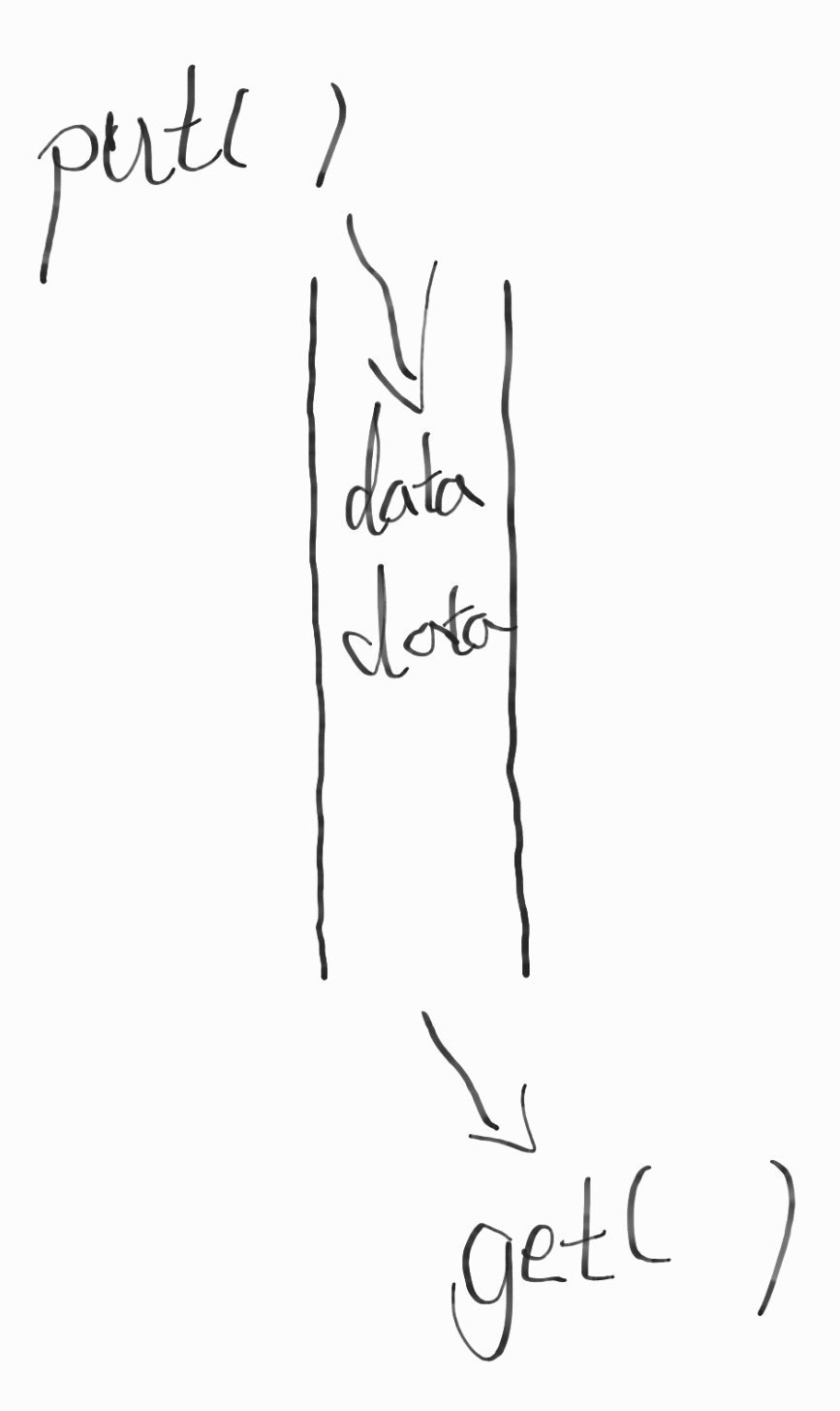# Python的并发编程（三）-线程间通信

### Queue队列

1. 创建Queue实例，Queue实例会被所有的线程共享。
2. put()添加元素
3. get()获取元素``````import time
from queue import Queue

def producer(out_q):
for i in range(10):
out_q.put(i)
time.sleep(2)

def consumer(in_q):
while True:
data = in_q.get()
print(data)

q = Queue()
t1.start()
t2.start()
``````

Queue实例已经拥有了所有需要的锁，所以他们可以安全的在任意多线程之间共享。

``````import time
from queue import Queue

_sentinel = object()

def producer(out_q):
for i in range(10):
out_q.put(i)
time.sleep(2)
out_q.put(_sentinel)

def consumer(in_q):
while True:
data = in_q.get()
if data is _sentinel:
in_q.put(_sentinel)
break
print(data)

q = Queue()
t1.start()
t2.start()
``````

``````import heapq

class PriorityQueue(object):
def __init__(self):
self._queue = []
self._count = 0

def put(self, item, priority):
while self._cv:
heapq.heappush(self._queue, (-priority, self._count, item))
self._count += 1
self._cv.notify()

def get(self):
with self._cv:
while len(self._queue) == 0:
self._cv.wait()
return heapq.heappop(self._queue)[-1]
``````

`q.join()`会等待队列中所有的信息被消费。

Queue对象的`put()``get()`都支持非阻塞和超时机制。

``````import queue

q = queue.Queue()

try:
data = q.get(block=False)
except queue.Empty:
pass

try:
q.put("item", block=False)
except queue.Full:
pass

try:
data = q.get(timeout=1)
except queue.Empty:
pass
``````